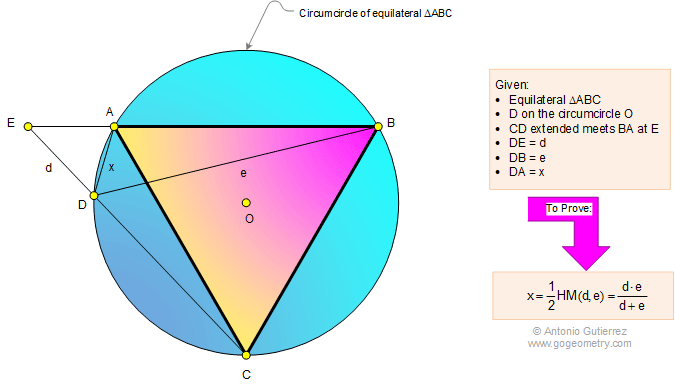# Geometry Problem 1126: Equilateral Triangle, Circumcircle, Metric Relation, Harmonic Mean, Similarity. Level: High School, SAT Prep, Honors Geometry, College, Math Education

< PREVIOUS PROBLEM  |  NEXT PROBLEM >

 The figure below shows an equilateral triangle ABC and a point D on the circumcircle O so that CD extended meets BA at E. If DE = d, DB = e, and DA = x, prove that x = one-half the harmonic mean of d and e, that is.Selected Topics

Home | SearchGeometry | Problems | All Problems | Open Problems | Visual Index | 10 Problems | 1121-1130 | Triangle | Equilateral triangle | Circumcircle | Harmonic Mean | Similarity | Email | Solution / comment.
Last updated: Jul 2, 2015 by Antonio Gutierrez.